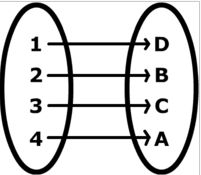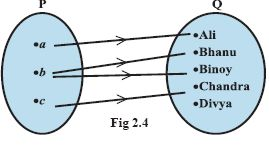kwizNET Subscribers, please login to turn off the Ads!
 Email us to get an instant 20% discount on highly effective K-12 Math & English kwizNET Programs!

#### Online Quiz (WorksheetABCD)

Questions Per Quiz = 2 4 6 8 10

### High School Mathematics - 24.10 Introduction to Functions

 Function Definition: A function is a relation that has exactly one "output" for every "input". Consider two sets A and B to form the Cartesian product. This Cartesian product forms the relations. Among these relations we select those relations that satisfy the condition - every element on A is related to only one element in set B. When a relation satisfies this rule we call it function. Function: Any relation on A x B in which in which every element in set A has a corresponding element on set B. It is denoted as f: A ->B or f: x -> f(x) or y = f(x) where y is a function of x. First element is also called domain, abscissa, preimage or the first component. Second element is also called range, ordinate, image or second component. A function can be represented by arrow diagram, set-builder notation, Cartesian form. Representation of a function Arrow DiagramDomain = {1,2,3,4} Range = {D,B,C,A} Set-builder Notation f = {(x,y)ly = 3x+5} Cartesian Form f = {(1,2), (2,3), (4,5)} Directions: Solve the following problems. Also write at least 5 examples of your own.
 Q 1: Let X = {1,2,3,4}. Determine whether r not each relation is a function from X to X.g = {(3,1), (4,2), (1,1)}h = {(2,1),(4,4), (3,4)}f = {(2,3), (1,4), (2,1), (3,2), (4,4)} Q 2: Given that f(x) = 2x and f: N->N, find the range f .Answer: Q 3: If A = {a, b, c} and B = {1,2}then the possible number of mappings from A to B is 468 Q 4: Let f: N -> N. If f = {(1,3), (2,5), (3,7)......}, find f(x)f(x) = 2x -1f(x) = 3x -2f(x) = 2x+1 Q 5: Which of the following relations are functions?y < x+3y > x + 5y = 3x+2 Q 6: Which of the following relations are functions?{(1,2), (1,3), (3,4), (4,5)}{(2,-3),(3,-4), (3,9), (5,8)}{(1,2), (3,7), (4,-6), (8,11)} Q 7: The given mapping is not a function. State whether true or falseTrueFalse Q 8: Write the domain of h: x-> 2/(x-7)Domain is set of all natural numbersDomain is all numbers except 7Domain is set of all real numbers Question 9: This question is available to subscribers only! Question 10: This question is available to subscribers only!

#### Subscription to kwizNET Learning System offers the following benefits:

• Unrestricted access to grade appropriate lessons, quizzes, & printable worksheets
• Instant scoring of online quizzes
• Progress tracking and award certificates to keep your student motivated
• Unlimited practice with auto-generated 'WIZ MATH' quizzes
• Child-friendly website with no advertisements
• Choice of Math, English, Science, & Social Studies Curriculums
• Excellent value for K-12 and ACT, SAT, & TOEFL Test Preparation
• Get discount offers by sending an email to discounts@kwiznet.com

 Quiz Timer